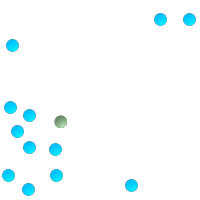## Name

ST_Centroid — Returns the geometric center of a geometry.

## Synopsis

`geometry ST_Centroid(`geometry g1`)`;

`geography ST_Centroid(`geography g1, boolean use_spheroid=true`)`;

## Description

Computes the geometric center of a geometry, or equivalently, the center of mass of the geometry as a `POINT`. For [`MULTI`]`POINT`s, this is computed as the arithmetic mean of the input coordinates. For [`MULTI`]`LINESTRING`s, this is computed as the weighted length of each line segment. For [`MULTI`]`POLYGON`s, "weight" is thought in terms of area. If an empty geometry is supplied, an empty `GEOMETRYCOLLECTION` is returned. If `NULL` is supplied, `NULL` is returned. If `CIRCULARSTRING` or `COMPOUNDCURVE` are supplied, they are converted to linestring wtih CurveToLine first, then same than for `LINESTRING`

New in 2.3.0 : support `CIRCULARSTRING` and `COMPOUNDCURVE` (using CurveToLine)

Availability: 2.4.0 support for geography was introduced.

The centroid is equal to the centroid of the set of component Geometries of highest dimension (since the lower-dimension geometries contribute zero "weight" to the centroid).This method implements the OpenGIS Simple Features Implementation Specification for SQL 1.1.This method implements the SQL/MM specification. SQL-MM 3: 8.1.4, 9.5.5

## Examples

In each of the following illustrations, the green dot represents the centroid of the source geometry.Centroid of a `MULTIPOINT`Centroid of a `LINESTRING`Centroid of a `POLYGON`Centroid of a `GEOMETRYCOLLECTION`
```SELECT ST_AsText(ST_Centroid('MULTIPOINT ( -1 0, -1 2, -1 3, -1 4, -1 7, 0 1, 0 3, 1 1, 2 0, 6 0, 7 8, 9 8, 10 6 )'));
st_astext
------------------------------------------
POINT(2.30769230769231 3.30769230769231)
(1 row)

SELECT ST_AsText(ST_centroid(g))
FROM  ST_GeomFromText('CIRCULARSTRING(0 2, -1 1,0 0, 0.5 0, 1 0, 2 1, 1 2, 0.5 2, 0 2)')  AS g ;
------------------------------------------
POINT(0.5 1)

SELECT ST_AsText(ST_centroid(g))
FROM  ST_GeomFromText('COMPOUNDCURVE(CIRCULARSTRING(0 2, -1 1,0 0),(0 0, 0.5 0, 1 0),CIRCULARSTRING( 1 0, 2 1, 1 2),(1 2, 0.5 2, 0 2))' ) AS g;
------------------------------------------
POINT(0.5 1)

```